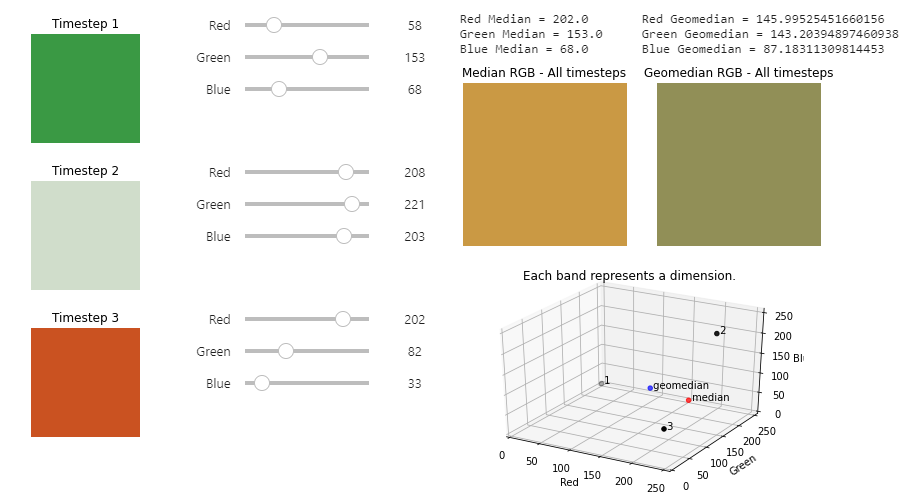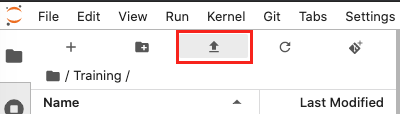# Geomedian widget¶

Geomedian composites are introduced in Session 3. Geomedians are a method of combining multiple timesteps of data into one image.

Geomedians were defined as follows:

A geomedian composite finds the median values of the bands for each pixel when considered together. This means they represent the data better than median composites.

This gives rise to a few common questions.

• What does it mean to consider the bands together?

• What might that look like?

• How does it affect the colour of each pixel in my final composite image?

To explore the answers to these questions, we can use an interactive module known as a widget. Instead of looking at one whole image, the widget focuses on a single pixel.

The geomedian widget gives three timesteps of data. You can click on the sliders to change the data for each timestep, which affects the pixel colour. In turn, this will impact the computation of the median and geomedian. As you will see, they are not always the same!An example of what the geomedian widget looks like. To interact with the widget, download and run the geomedian widget notebook.

Follow the instructions below to download the widget notebook. It can help with understanding the following concepts:

• In which cases are the median and geomedian very similar or the same?

• In which cases are the median and geomedian very different?

• Why are geomedians more representative of the whole dataset, compared to medians?

## Download geomedian widget notebook¶

Download the geomedian widget notebook

To view this notebook on the Sandbox, you will need to first download it to your computer, then upload it to the Sandbox. Follow these instructions:

1. Download the notebook by clicking the link above.

2. On the Sandbox, open the Training folder.

3. Click the Upload Files button as shown below.1. Select the downloaded notebook using the file browser. Click OK.

2. The solution notebook will appear in the Training folder. Double-click to open it.